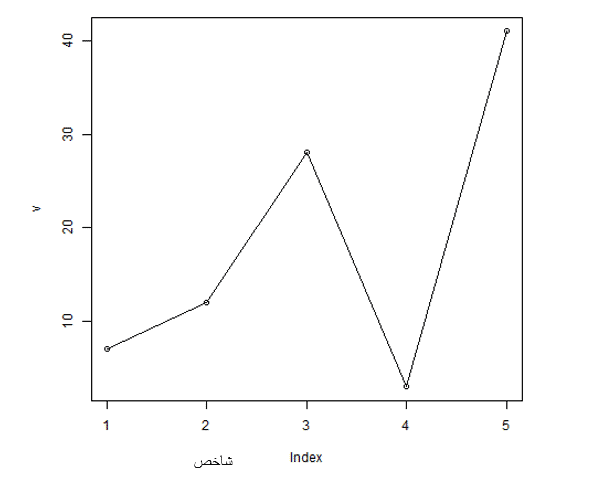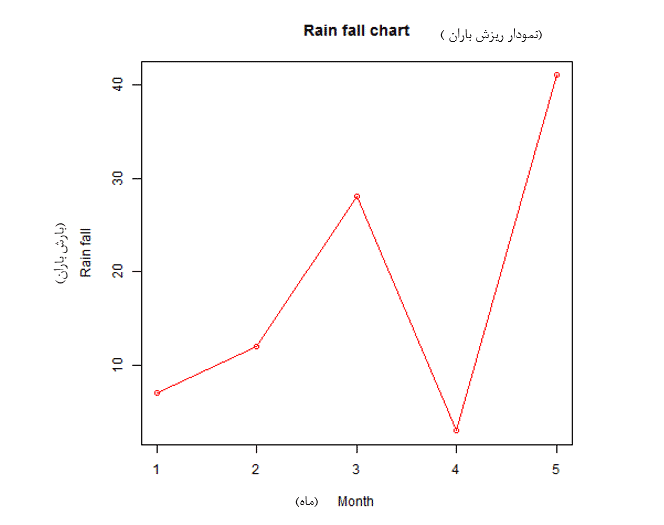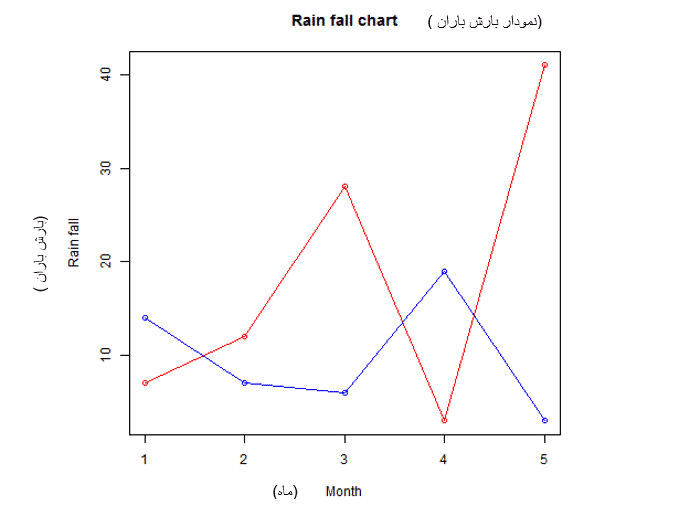# blog posts# A line chart; It is a diagram that connects a series of points by drawing lines between them. These points are usually arranged according to one of their coordinates (usually x coordinates).

Line charts are commonly used to determine the flow of data.

Plot () function in r; Used to create line charts.

## Syntax

The basic syntax for creating linear diagrams in the R programming language is as follows:

plot (v, type, col, xlab, ylab)

Variables used in the above coding; as follows:

• v is a vector that contains numerical variables.
• type adopts the variable “p” only to draw points; It takes “l” to draw lines and “o” to draw points and lines.
• xlab is the name of the x-axis.
• ylab is the name of the y axis.
• main Specifies the title of the chart.
• col is used to represent the color of dots and lines.

## Example

A simple bar graph is created using input vectors and typical parameters such as “O”. Script below; Creates and stores a bar chart in the currently working directory in R.

# Create the data for the chart.

v <- c (7,12,28,3,41)

# Give the chart file a name.

png (file = “line_chart.jpg”)

# Plot the bar chart.

plot (v, type = “o”)

# Save the file.

dev.off ()

When we run the above code; We get the following result:## lable; Color and title of line chart

The properties of linear graphs can be expanded using additional parameters. We add color to points and lines; Assign a title to the chart and add labels to the x and y axes.

#### Example

# Create the data for the chart.

v <- c (7,12,28,3,41)

# Give the chart file a name.

png (file = “line_chart_label_colored.jpg”)

# Plot the bar chart.

plot (v, type = “o”, col = “red”, xlab = “Month”, ylab = “Rain fall”,

main = “Rain fall chart”)

# Save the file.

dev.off ()

When we run the following code; The following result will obtain:(Rainfall)

## Several lines in a line chart

Using the lines () function, more than one line can also be drawn in a diagram. After the first line is drawn; The lines () function can use an additional vector as input to plot the second line in the graph.

# Create the data for the chart.

v <- c (7,12,28,3,41)

t <- c (14,7,6,19,3)

# Give the chart file a name.

png (file = “line_chart_2_lines.jpg”)

# Plot the bar chart.

plot (v, type = “o”, col = “red”, xlab = “Month”, ylab = “Rain fall”,

main = “Rain fall chart”)

lines (t, type = “o”, col = “blue”)

# Save the file.

dev.off ()

When we run the above code; We get the following result: